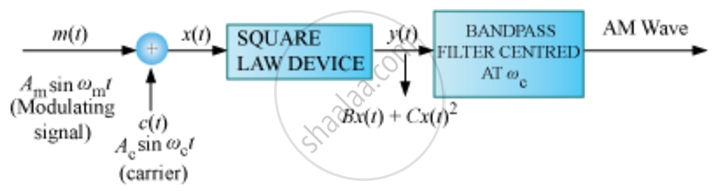# Explain the Process of Amplitude Modulation. - Physics

Explain the process of amplitude modulation.

#### Solution

Amplitude Modulation: Amplitude modulation is produced by varying the amplitude of the carrier waves in accordance with the amplitude of the modulating wave.

Let the carrier wave be c(t) = Acsinωct and the modulating signal be m(t) = Amsinωmwhere ω= 2πfm is the angular frequency of the message signal.

Modulated signal cm(t) is

cm(t)=(Ac+Am sinωmt)sinωct

where μ = Am/Ais the modulation index.

c_m(t)=A_csinomega_ct+(muA_c)/2cos(omega_c-omega_m)t-(muA_c)/2cos(omega_c+omega_m)t

ωc - ωm and ωcm are the lower side and upper side frequencies, respectively.

Production of Amplitude Modulated WaveConcept: Amplitude Modulation
Is there an error in this question or solution?